Next: 3.2.2 Multi Scale Method Up: 3.2 Formalism Previous: 3.2 Formalism

## 3.2.1 DCA

The DCA is a systematic quantum cluster theory that maps the lattice problem onto a self-consistently embedded cluster problem. It is an extension of the dynamical mean-field theory (DMFT)(6,5) which systematically incorporates non-local correlations. In the limit when the cluster size is one (i.e. single site), it recovers the purely local DMFT solution, systematically incorporates non-local corrections as the cluster size increases, and finally becomes exact when the cluster size equals the size of the lattice.

The respective approximations for the DMFT and DCA may be derived by approximating the Laue function which describes momentum conservation at the vertices of the irreducible diagrams:(3.2)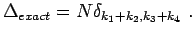(3.3)

In the DMFT, the Laue function is approximated with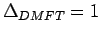for all combinations of,,and. In doing so, all electron propagators in the self-energy diagrams may be averaged over the first Brillouin Zone (BZ); thus relinquishing any momentum dependence of the self-energy. Hence, the DMFT lattice Green's function contains local correlations of the system but is unable to capture non-local correlations. The DCA sets out to systematically include these non-local contributions. This is accomplished by partially restoring momentum conservation of the irreducible vertices. We divide the BZ intoidentical discrete sub-cells as illustrated in Fig. 3.1. The center of each cell is labeled by, and the surrounding points by, so that any arbitrary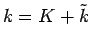. In the DCA, this partial momentum conservation is expressed by the Laue function:(3.4)Therefore, all propagators may freely be summed over intra-cell momenta, yielding the coarse grained Green's function(3.5)

In so doing, only momentum conservation of magnitudeis neglected, while larger inter-cell transfers are preserved. The resulting self-energy diagrams are now those of a finite cluster of sizewhere each lattice propagator has been replaced by its coarse-grained analog, and the remaining cluster problem is defined by. We can write for the DCA lattice Green's function(3.6)

where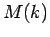is a function which maps momentumresiding in a certain sub-cell of the BZ to its cluster momentumand the lattice self-energy is approximated by that of the cluster problem.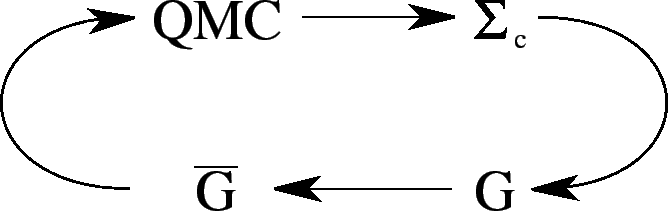The remaining embedded cluster problem must be solved with a self-consistency requirement that the Green's function calculated on the cluster. Fig. 3.2 depicts the corresponding DCA algorithm: Starting with an initial guess for the self-energy, we construct the coarse-grained Green's function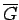from the corresponding lattice(see Eq. 3.5). In the next step, we utilize one of the many available cluster solvers to determine the cluster self-energy. This is the numerically most involved step and a variety of numerical techniques may be applied. At this point, we use the new estimate for the self-energy to re-initialize the self-consistency loop. It is important to notice that in this procedure only the irreducible lattice quantities are approximated by their cluster equivalent - i.e. the self-energy.

The DCA has been successfully implemented with a variety of cluster solvers of which some are exact but limited in cluster size, while others are applicable up to larger length-scales but involve varying degrees of approximation. Some of the cluster solvers which have been used in conjunction with the DCA include the non-crossing approximation (NCA)(7), the fluctuation exchange approximation (FLEX)(3,8) and the Quantum Monte Carlo (QMC)(2) method. While NCA and FLEX involve various levels of approximations, QMC is of special interest since it provides an essentially numerically exact solution to the problem. Although the QMC constitutes a precise cluster solver, it becomes prohibitively expensive for large clusters. The range of applicability of exact calculations is thus restricted to relatively short length-scales. However, various properties of strongly-correlated systems are not accounted for (e.g. Mermin-Wagner theorem(9)) due to the absence of long-ranged fluctuations in these solutions.Next: 3.2.2 Multi Scale Method Up: 3.2 Formalism Previous: 3.2 Formalism
© Cyrill Slezak